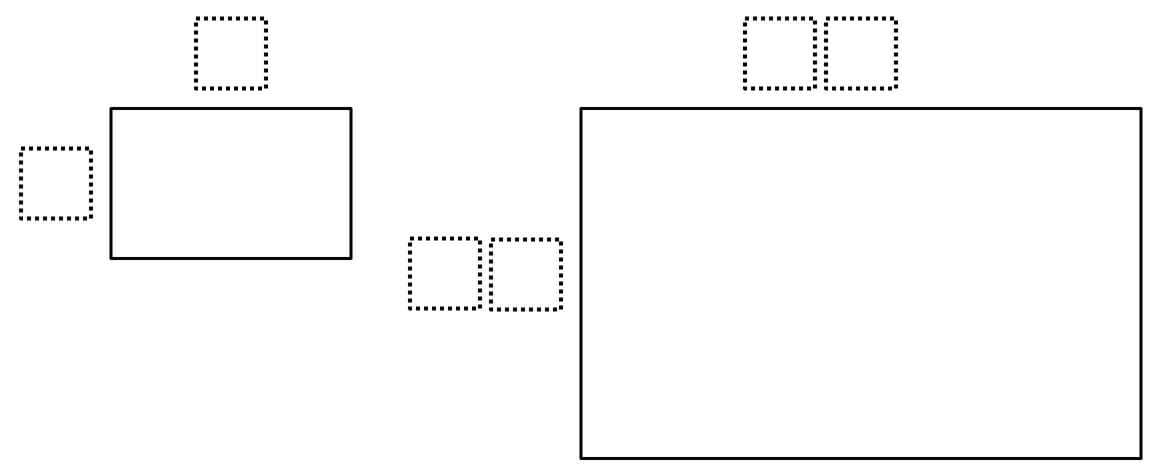Home > Grade 7 > Geometry > Similar Shapes

# Similar Shapes

Directions: Using the digits 0-9, at most one time each, fill in the boxes so that one rectangle is a scaled drawing of the other.### Hint

Enlargements occur when the scale factor is greater than 1.

How many times bigger is the second rectangle compared to the first rectangle?

There are many answers. Here are two of them:

First rectangle is 2 x 8
Second rectangle is 14 x 56
Scale factor would be 7.

First rectangle is 3 x 8
Second rectangle is 15 x 40.
Scale factor would be 5.

Source: Gian Cavaliere

## Sides of a Triangle

Directions: The perimeter of a triangle is 20 units. Using whole numbers, how many sets …

1.How is the answer 3×8 and 15×40 with scale factor 5 following the directions? Are we allowed to use two digit numbers?

•Sure you can! You can adapt and change the question in any way you want. Maybe only allow single digits at first and then if they are able to complete that, allow them to try two and even three digit numbers.

•Sorry for the confusion. Yes, two-digit numbers are allowed. The problem has been updated to reflect that.

2.I love Open Middle Problems, but I think I am missing something. Is there a way to use the template provided for problems like this so that the students can actually type inside the box?

•Great question, Kelly. Unfortunately to make the problems interactive would require more sophisticated programming than what is offered through WordPress.
What I sometimes do is click on the image, then right-click to copy it. I then paste it into a document that students can mark up, then explain their strategy. If several answers are possible, I paste it a few times.
Some educators have adapted Open Middle problems into Desmos to make them interactive. For example: https://teacher.desmos.com/collection/5e8d1d887bb53c0935384eca, but others can be find by Googling.

3.first rectangle like 14×19=266